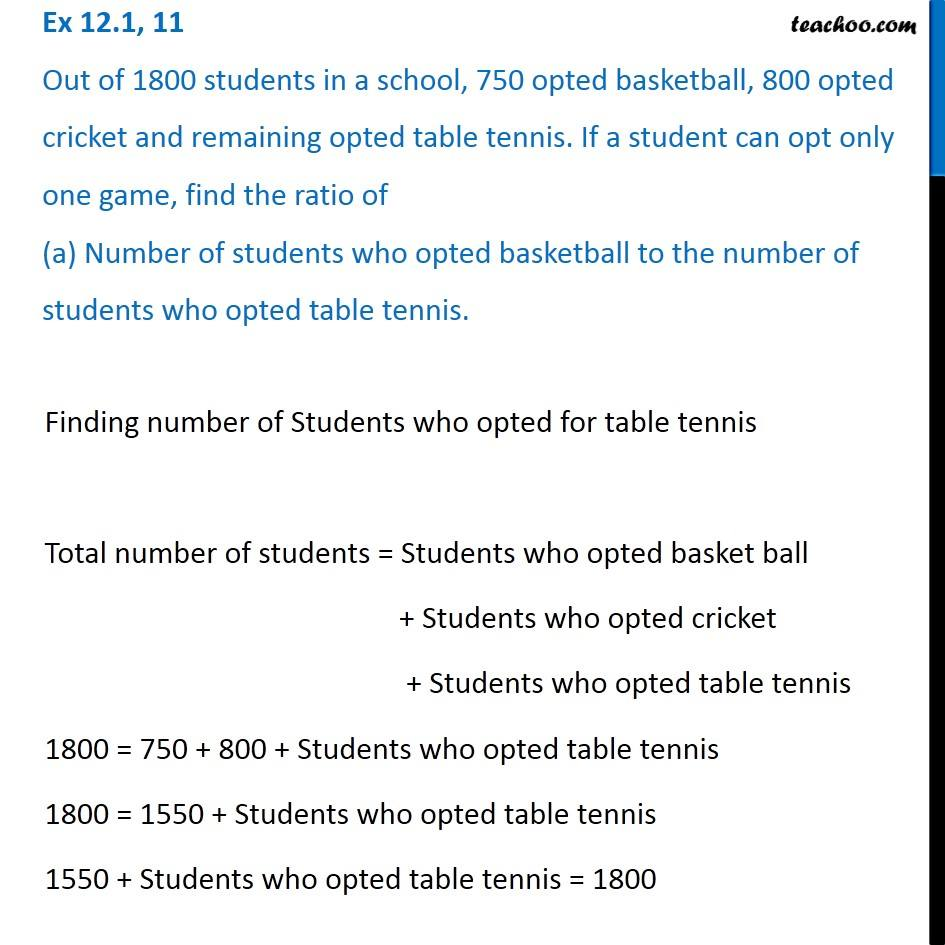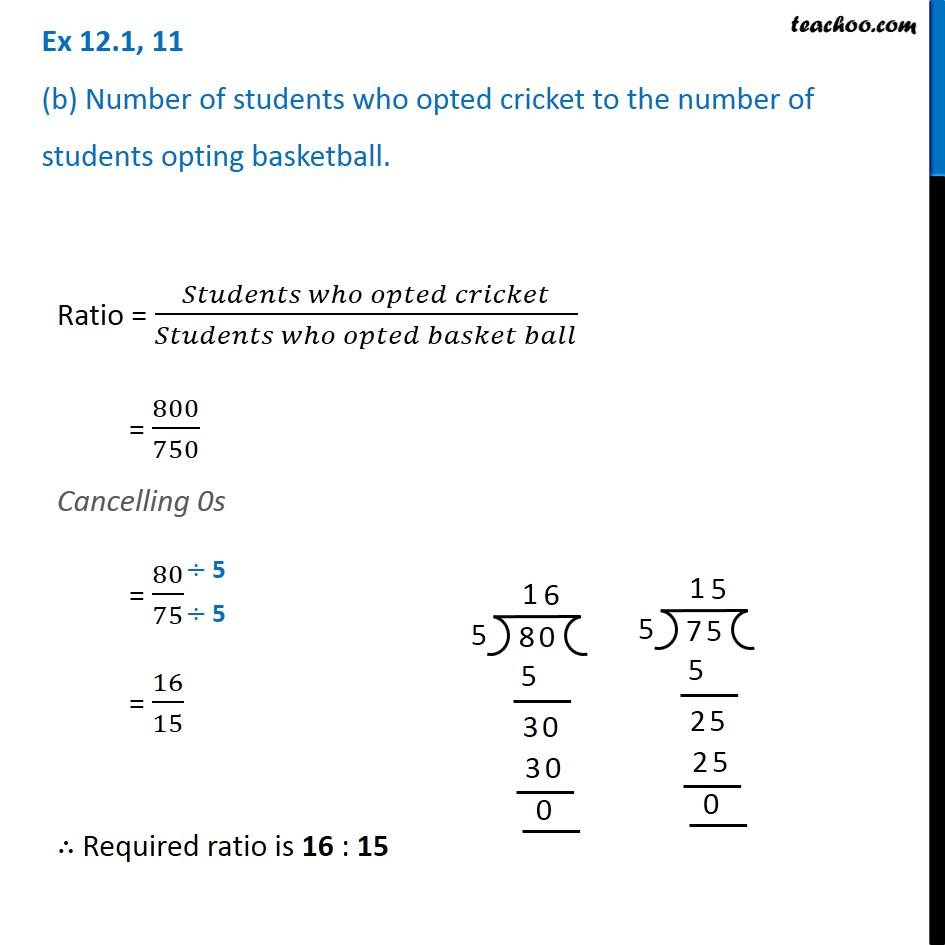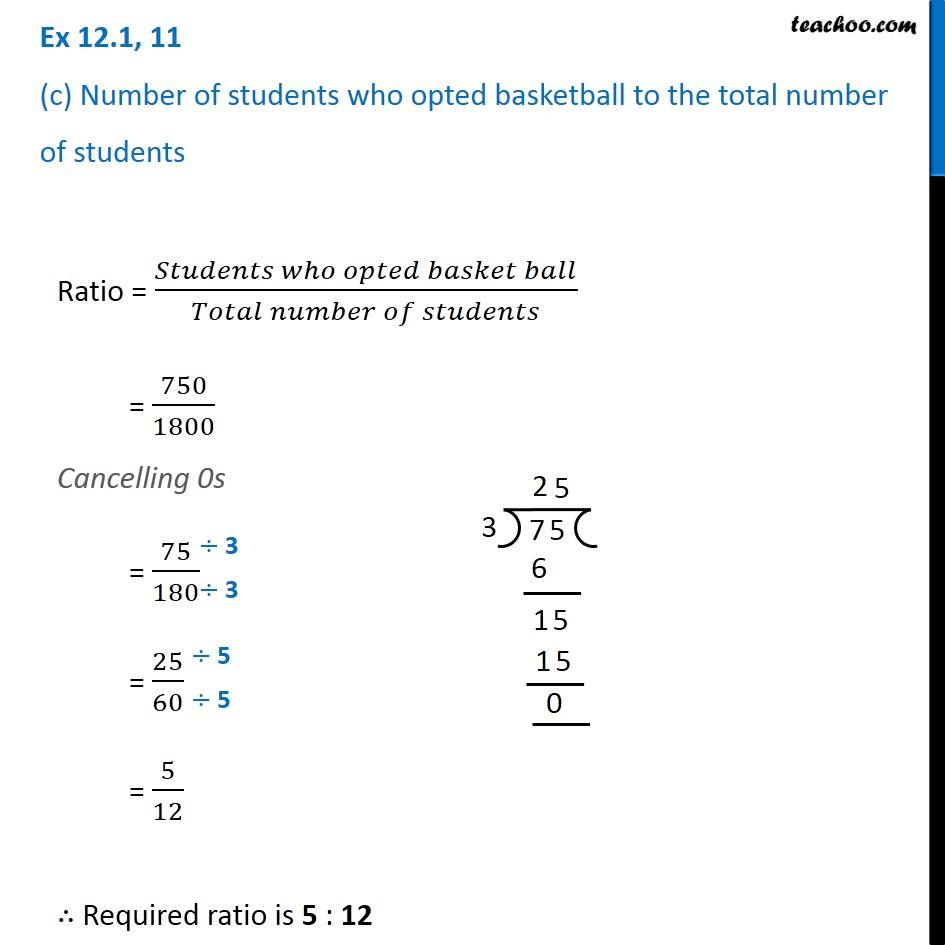Ratios

Chapter 12 Class 6 Ratio and Proportion
Concept wiseLearn in your speed, with individual attention - Teachoo Maths 1-on-1 Class

### Transcript

Ex 12.1, 11 Out of 1800 students in a school, 750 opted basketball, 800 opted cricket and remaining opted table tennis. If a student can opt only one game, find the ratio of (a) Number of students who opted basketball to the number of students who opted table tennis.Finding number of Students who opted for table tennis Total number of students = Students who opted basket ball + Students who opted cricket + Students who opted table tennis 1800 = 750 + 800 + Students who opted table tennis 1800 = 1550 + Students who opted table tennis 1550 + Students who opted table tennis = 1800 Students who opted table tennis = 1800 − 1550 Students who opted table tennis = 250 Ratio = (𝑆𝑡𝑢𝑑𝑒𝑛𝑡𝑠 𝑤ℎ𝑜 𝑜𝑝𝑡𝑒𝑑 𝑏𝑎𝑠𝑘𝑒𝑡 𝑏𝑎𝑙𝑙)/(𝑆𝑡𝑢𝑑𝑒𝑛𝑡𝑠 𝑤ℎ𝑜 𝑜𝑝𝑡𝑒𝑑 𝑡𝑎𝑏𝑙𝑒 𝑡𝑒𝑛𝑛𝑖𝑠) = 750/250 Cancelling 0s = 75/25 = 15/5 = 3/1 ∴ Required ratio is 3 : 1 Ex 12.1, 11 (b) Number of students who opted cricket to the number of students opting basketball.Ratio = (𝑆𝑡𝑢𝑑𝑒𝑛𝑡𝑠 𝑤ℎ𝑜 𝑜𝑝𝑡𝑒𝑑 𝑐𝑟𝑖𝑐𝑘𝑒𝑡)/(𝑆𝑡𝑢𝑑𝑒𝑛𝑡𝑠 𝑤ℎ𝑜 𝑜𝑝𝑡𝑒𝑑 𝑏𝑎𝑠𝑘𝑒𝑡 𝑏𝑎𝑙𝑙) = 800/750 Cancelling 0s = 80/75 = 16/15 ∴ Required ratio is 16 : 15 Ex 12.1, 11 (c) Number of students who opted basketball to the total number of studentsRatio = (𝑆𝑡𝑢𝑑𝑒𝑛𝑡𝑠 𝑤ℎ𝑜 𝑜𝑝𝑡𝑒𝑑 𝑏𝑎𝑠𝑘𝑒𝑡 𝑏𝑎𝑙𝑙)/(𝑇𝑜𝑡𝑎𝑙 𝑛𝑢𝑚𝑏𝑒𝑟 𝑜𝑓 𝑠𝑡𝑢𝑑𝑒𝑛𝑡𝑠) = 750/1800 Cancelling 0s = 75/180 = 25/60 = 5/12 ∴ Required ratio is 5 : 12